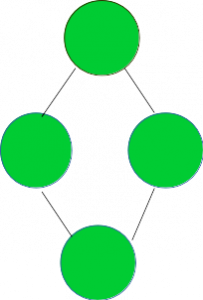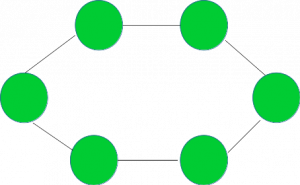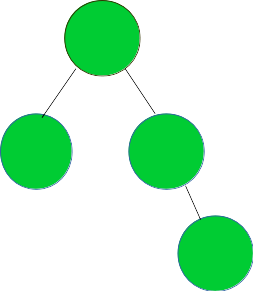# Check if the given graph represents a Ring Topology

Given a graph G, the task is to check if it represents a Ring Topology.

A Ring Topology is the one shown in the image below:Examples:

```Input :  Graph =Output : YES

Input : Graph =Output : NO
```

## Recommended: Please try your approach on {IDE} first, before moving on to the solution.

A graph of V vertices represents a Ring topology if it satisfies the following three conditions:

1. Number of vertices >= 3.
2. All vertices should have degree 2.
3. No of edges = No of Vertices.

The idea is to traverse the graph and check if it satisfies the above three conditions. If yes, then it represents a Ring Topology otherwise not.

Below is the implementation of the above approach:

## C++

 `// CPP program to check if the given graph ` `// represents a Ring topology ` ` `  `#include ` `using` `namespace` `std; ` ` `  `// A utility function to add an edge in an ` `// undirected graph. ` `void` `addEdge(vector<``int``> adj[], ``int` `u, ``int` `v) ` `{ ` `    ``adj[u].push_back(v); ` `    ``adj[v].push_back(u); ` `} ` ` `  `// A utility function to print the adjacency list ` `// representation of graph ` `void` `printGraph(vector<``int``> adj[], ``int` `V) ` `{ ` `    ``for` `(``int` `v = 0; v < V; ++v) { ` `        ``cout << ``"\n Adjacency list of vertex "` `             ``<< v << ``"\n head "``; ` `        ``for` `(``auto` `x : adj[v]) ` `            ``cout << ``"-> "` `<< x; ` `        ``printf``(``"\n"``); ` `    ``} ` `} ` ` `  `/* Function to return true if the graph represented  ` `   ``by the adjacency list represents a Ring topology  ` `   ``else return false */` `bool` `checkRingTopologyUtil(vector<``int``> adj[], ``int` `V, ``int` `E) ` `{ ` `    ``// Number of edges should be equal ` `    ``// to Number of vertices ` `    ``if` `(E != V) ` `        ``return` `false``; ` ` `  `    ``// For a graph to represent a ring topology should have ` `    ``// greater than 2 nodes ` `    ``if` `(V <= 2) ` `        ``return` `false``; ` ` `  `    ``int``* vertexDegree = ``new` `int``[V + 1]; ` `    ``memset``(vertexDegree, 0, ``sizeof` `vertexDegree); ` ` `  `    ``// calculate the degree of each vertex ` `    ``for` `(``int` `i = 1; i <= V; i++) { ` `        ``for` `(``auto` `v : adj[i]) { ` `            ``vertexDegree[v]++; ` `        ``} ` `    ``} ` ` `  `    ``// countDegree2 stores the count of ` `    ``// the vertices having degree 2 ` `    ``int` `countDegree2 = 0; ` ` `  `    ``for` `(``int` `i = 1; i <= V; i++) { ` `        ``if` `(vertexDegree[i] == 2) { ` `            ``countDegree2++; ` `        ``} ` `    ``} ` ` `  `    ``// if all three necessary conditions as discussed, ` `    ``// satisfy return true ` `    ``if` `(countDegree2 == V) { ` `        ``return` `true``; ` `    ``} ` `    ``else` `{ ` `        ``return` `false``; ` `    ``} ` `} ` ` `  `// Function to check if the graph represents a Ring topology ` `void` `checkRingTopology(vector<``int``> adj[], ``int` `V, ``int` `E) ` `{ ` `    ``bool` `isRing = checkRingTopologyUtil(adj, V, E); ` `    ``if` `(isRing) { ` `        ``cout << ``"YES"` `<< endl; ` `    ``} ` `    ``else` `{ ` `        ``cout << ``"NO"` `<< endl; ` `    ``} ` `} ` ` `  `// Driver code ` `int` `main() ` `{ ` `    ``// Graph 1 ` `    ``int` `V = 6, E = 6; ` `    ``vector<``int``> adj1[V + 1]; ` `    ``addEdge(adj1, 1, 2); ` `    ``addEdge(adj1, 2, 3); ` `    ``addEdge(adj1, 3, 4); ` `    ``addEdge(adj1, 4, 5); ` `    ``addEdge(adj1, 6, 1); ` `    ``addEdge(adj1, 5, 6); ` `    ``checkRingTopology(adj1, V, E); ` ` `  `    ``// Graph 2 ` `    ``V = 5, E = 4; ` `    ``vector<``int``> adj2[V + 1]; ` `    ``addEdge(adj2, 1, 2); ` `    ``addEdge(adj2, 1, 3); ` `    ``addEdge(adj2, 3, 4); ` `    ``addEdge(adj2, 4, 5); ` `    ``checkRingTopology(adj2, V, E); ` ` `  `    ``return` `0; ` `} `

## Python3

 `# Python3 program to check if the given graph ` `# represents a star topology ` ` `  `# A utility function to add an edge in an ` `# undirected graph. ` ` `  `def` `addEdge(adj, u, v): ` `    ``adj[u].append(v) ` `    ``adj[v].append(u) ` ` `  `# A utility function to prthe adjacency list ` `# representation of graph ` `def` `printGraph(adj, V): ` ` `  `    ``for` `v ``in` `range``(V): ` `        ``print``(``"Adjacency list of vertex "``,v,``"\n head "``) ` `        ``for` `x ``in` `adj[v]: ` `            ``print``(``"-> "``,x,end``=``" "``) ` `        ``printf() ` ` `  `# /* Function to return true if the graph represented ` `#    by the adjacency list represents a ring topology ` `#    else return false */ ` `def` `checkRingTopologyUtil(adj, V, E): ` ` `  `    ``# Number of edges should be equal ` `    ``# to (Number of vertices - 1) ` `    ``if` `(E !``=` `(V)): ` `        ``return` `False` ` `  `    ``# For a graph to represent a ring topology should have ` `    ``# greater than 2 nodes ` `    ``if` `(V <``=` `2``): ` `        ``return` `False` ` `  `    ``vertexDegree ``=` `[``0``]``*``(V ``+` `1``) ` ` `  `    ``# calculate the degree of each vertex ` `    ``for` `i ``in` `range``(V``+``1``): ` `        ``for` `v ``in` `adj[i]: ` `            ``vertexDegree[v] ``+``=` `1` `     `  `    ``# countDegree2 stores the count of ` `    ``# the vertices having degree 2 ` `    ``countDegree2 ``=` `0` ` `  `    ``for` `i ``in` `range``(``1``, V ``+` `1``): ` `        ``if` `(vertexDegree[i] ``=``=` `2``): ` `            ``countDegree2 ``+``=` `1` ` `  `    ``# if all three necessary conditions as discussed, ` `    ``# satisfy return true ` `    ``if` `(countDegree2 ``=``=` `V): ` `        ``return` `True` `    ``else``: ` `        ``return` `False` ` `  `# Function to check if the graph represents a ring topology ` `def` `checkRingTopology(adj, V, E): ` ` `  `    ``isRing ``=` `checkRingTopologyUtil(adj, V, E) ` `    ``if` `(isRing): ` `        ``print``(``"YES"``) ` ` `  `    ``else``: ` `        ``print``(``"NO"` `) ` ` `  `# Driver code ` ` `  `# Graph 1 ` `V,E ``=` `6``,``6` `adj1 ``=` `[[] ``for` `i ``in` `range``(V ``+` `1``)] ` `addEdge(adj1, ``1``, ``2``) ` `addEdge(adj1, ``2``, ``3``) ` `addEdge(adj1, ``3``, ``4``) ` `addEdge(adj1, ``4``, ``5``) ` `addEdge(adj1, ``6``, ``1``) ` `addEdge(adj1, ``5``, ``6``) ` `checkRingTopology(adj1, V, E) ` ` `  `# Graph 2 ` `V,E ``=` `5``,``4` `adj2 ``=` `[[] ``for` `i ``in` `range``(V ``+` `1``)] ` `addEdge(adj2, ``1``, ``2``) ` `addEdge(adj2, ``1``, ``3``) ` `addEdge(adj2, ``3``, ``4``) ` `addEdge(adj2, ``4``, ``2``) ` `checkRingTopology(adj2, V, E) ` ` `  `# This code is contributed by mohit kumar 29 `

Output:

```YES
NO
```

Time Complexity: O(V + E) where V and E are the numbers of vertices and edges in the graph respectively.My Personal Notes arrow_drop_upCheck out this Author's contributed articles.

If you like GeeksforGeeks and would like to contribute, you can also write an article using contribute.geeksforgeeks.org or mail your article to contribute@geeksforgeeks.org. See your article appearing on the GeeksforGeeks main page and help other Geeks.

Please Improve this article if you find anything incorrect by clicking on the "Improve Article" button below.

Improved By : mohit kumar 29